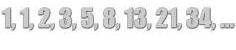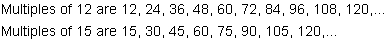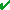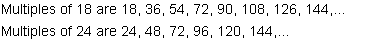Multiples and Least Common MultiplesThe Least Common Multiple is...

...the smallest multiple common to all whole numbers in the set.

Problem: An ice cream truck visits Jeannette's neighborhood every 4 days during the summer. Unfortunately, she missed it today. When can Jeannette expect the ice cream truck to visit her neighborhood again?

Solution: The ice cream truck will visit on days 4, 8, 12, 16, 20, 24, 28, 32, ...

In the problem above, we found multiples of the whole number 4. The multiples of a whole number are found by taking the product of any counting number and that whole number. For example, to find the multiples of 3, multiply 3 by 1, 3 by 2, 3 by 3, and so on. To find the multiples of 5, multiply 5 by 1, 5 by 2, 5 by 3, and so on. The multiples are the products of these multiplications. Some examples of multiples can be found below. In each example, the counting numbers 1 through 8 are used. However, the list of multiples for a whole number is endless. The ... at the end of each list below lets us know that the list really goes on forever.

 Example 1: Find the multiples of the whole number 4. Multiplication: 4 x 1 4 x 2 4 x 3 4 x 4 4 x 5 4 x 6 4 x 7 4 x 8 Multiples of 4: 4 8 12 16 20 24 28 32 Solution: The multiples of 4 are 4, 8, 12, 16, 20, 24, 28, 32,...
 Example 2: Find the multiples of the whole number 5. Multiplication: 5 x 1 5 x 2 5 x 3 5 x 4 5 x 5 5 x 6 5 x 7 5 x 8 Multiples of 5: 5 10 15 20 25 30 35 40 Solution: The multiples of 5 are 5, 10, 15, 20, 25, 30, 35, 40,...
 Example 3: Find the multiples of the whole number 7. Multiplication: 7 x 1 7 x 2 7 x 3 7 x 4 7 x 5 7 x 6 7 x 7 7 x 8 Multiples of 7: 7 14 21 28 35 42 49 56 Solution: The multiples of 7 are 7, 14, 21, 28, 35, 42, 49, 56,...Problem: During the summer months, one ice cream truck visits Jeannette's neighborhood every 4 days and another ice cream truck visits her neighborhood every 5 days. If both trucks visited today, when is the next time both trucks will visit on the same day?

Truck               Days of Visits

1                      4, 8, 12, 16, 20, 24, 28, 32, 36, 40, 44,...

2                      5, 10, 15, 20, 25, 30, 35, 40, 45, 50, 55,...

Solution: Both ice cream trucks will visit Jeannette's neighborhood in 20 days and in 40 days. However, the problem asks: when is the next time both trucks will visit on the same day?, so the final answer is IN 20 DAYS.

In the problem above, we have found multiples of the numbers 4 and 5. We have also found the Least Common Multiple (LCM) of 4 and 5, which is 20.

To find the Least Common Multiple of two or more whole numbers, follow this procedure:

1. Make a list of multiples for each whole number.
2. Continue your list until at least two multiples are common to all lists.
3. Identify the common multiples.
4. The Least Common Multiple (LCM) is the smallest of these common multiples.

In Examples 4 and 5, place your mouse over the lists of multiples to see the common multiples.

Example 4: Find the LCM of 12 and 15.Common multiples of 12 and 15 are 60 and 120

The least common multiple of 12 and 15 is 60.

Solution: LCM = 60Example 5: Find the LCM of 18 and 24.Common multiples of 18 and 24 are 72 and 144

The least common multiple of 18 and 24 is 72.

Solution: LCM = 72Summary: The multiples of a whole number are found by taking the product of any counting number and that whole number. The Least Common Multiple (LCM) of a set of whole numbers is the smallest multiple common to all whole numbers in the set.

Exercises

Directions: Read each question below. Click once in an ANSWER BOX and type in your answer; then click ENTER. After you click ENTER, a message will appear in the RESULTS BOX to indicate whether your answer is correct or incorrect. To start over, click CLEAR.

 1 Find the LCM of 9 and 10. ANSWER BOX:  LCM =   RESULTS BOX:
 2 Find the LCM of 14 and 42. ANSWER BOX:  LCM =   RESULTS BOX:
 3 Find the LCM of 18 and 30. ANSWER BOX:  LCM =   RESULTS BOX:
 4 Find the LCM of 8, 9 and 12. ANSWER BOX:  LCM =   RESULTS BOX:
 5 Mrs. Hernandez waters one of her plants every 10 days and another plant every 14 days. If she waters both plants today, when is the next time both plants will be watered on the same day? ANSWER BOX:  LCM =   RESULTS BOX: